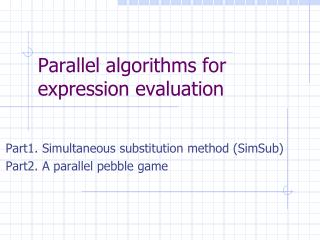DownloadDownload PresentationParallel algorithms for expression evaluation

# Parallel algorithms for expression evaluation

Télécharger la présentation## Parallel algorithms for expression evaluation

- - - - - - - - - - - - - - - - - - - - - - - - - - - E N D - - - - - - - - - - - - - - - - - - - - - - - - - - -
##### Presentation Transcript

1. Parallel algorithms for expression evaluation Part1. Simultaneous substitution method (SimSub) Part2. A parallel pebble game

2. Example 1: expression evaluation Summing the numbers 2, 1, 3, 2, 1, 3, 2, 1 we can write a sequential program x1 = 2; x2 = x1+1; x3 = x2+3; x4 = x3+2; x5 = x4+1; x6 = x5+3; x7 = x6+2; x8=x7+1

3. Simultaneous Substitutions Method (SimSub) • x = Expression(y) and y = Expression1 a variable y can be substituted by its expression or by its value if it is known (if Expression1 is a constant) • assume we have • a sequence of expressions defining variables, • each expression is of a constant size • variables are numbered and • i-th expression depends only on variables with numbers smaller than i e.g. x1=4 x2=x1+5 x3=x1+x2*7 x4=x2*x3

4. One parallel step: for each expression substitute its variables by their expression if it is safe; • safe means that corresponding expression has at most one variable • Example: summing the numbers 2, 1, 3, 2, 1, 3, 2, 1 we can write a sequential program x1 = 2; x2 = x1+1; x3 = x2+3; x4 = x3+2; x5 = x4+1; x6 = x5+3; x7 = x6+2 • after parallel step 1 x1 = 2; x2 = 3; x3 = x1+4; x4 = x2+5; x5 = x3+3; x6 = x4+4; x7 = x5+5 • after parallel step 2 x1 = 2; x2 = 3; x3 = 6; x4 = 8; x5 = x1+7; x6 = x2+9; x7 = x3+8 • after parallel step 2 x1 = 2; x2 = 3; x3 = 6; x4 = 8; x5 = 9; x6 = 12; x7 = 14 • output = sum = x7 In this way we can sum 1024 numbers in 10 rounds

5. Tree-contraction ======================================== Parallel algorithms related to expression evaluation correspond to parallel algorithmic technique called tree-contraction =============================================== • Tree-contraction is used in parallel expression evaluation • Since the structure of a expression is a tree there are different tree-contraction techniques • Basic operations are: - redirecting edges of the tree - removing nodes marking (pebbling) nodes - creating additional edges • the final aim is to guarantee that logarithmic number of contractions is sufficient

6. Tree-contraction related to SimSub algorithm

7. Example Substitutions method works in O(log n) time. In each iteration the active tree is reduced by 1/3 at least. The active tree consists of nodes relevant to the output computation, The root is the output variable (the last one).

8. Homework: is it good object for SimSub? Why? Fn is the smallest tree which needs n iterations in tree contraction related to SimSub algorithm

9. How the SimSub algorithm works for general graphs ? • sometimes very poor • (e.g. when computing Fibonacci numbers) • generally with n processors we need • n-1 iterations • only one processor really works • each time, so the same can be done with a single processor

10. SimSub will terminate after O(log (tree-size(graph)) iterations • for the dependency graph of Fibonacci numbers tree-size (number of paths) is exponential.

11. Other tree contraction method: parallel pebble game (PPG) • Parallel pebble game (PPG) works for full binary trees, full means each father has exactly 2 sons

12. Parallel pebble game on binary tree • Within the game each node v of the tree has associated with it similar node denoted by cond(v). • At the outset of the game cond(v)=v, for all v • During the game the pairs (v,cond(v)) can be thought of as additional edges • Node v is ”active” if and only if cond(v)v

13. Pebbling • Pebbling a node denotes the fact that in the current state of the game the processor associated with that node has sufficient information to evaluate the subtree rooted here

14. Three operationsactive, square and pebble Activate for all non-leaf nodes v in parallel do ifv is not active and precisely one of its sons is pebbledthen cond(v) becomes the other son ifv is not active and both sons are pebbledthen cond(v) becomes one of the sons arbitrarily Square for all nodes v in parallel do cond(v) cond(cond(v)) Pebble for all nodes v in parallel do if cond(v) is pebbled then pebble v

15. Key result • At the outset of the game only the leaves of the tree are pebbled. • One composite move of the pebbling game is the sequence of individual operations (activate, square, square, pebble) Theorem Let T be a binary tree with n leaves. If initially only the leaves are pebbled then after log2n moves of the pebbling game the root of T becomes pebbled.

16. Example square activate square pebbling

17. + 5 * 3 + * 3 2 2 The application of the pebbling game • Consider the arithmetic expression ((3+(2*2))*3+5) • We assign a processor to each non-leaf node of the tree.

18. + + + + 5 5 5 5 * * * * 3 3 3 3 + + + + * * * * 3 3 3 3 2 2 2 2 2 2 2 2 X+5 X*3 X+3 X*2 3x+5 3(2x+3)+5 3x+9 3(2x)+9 2x+3 2x+3 2x 2x

19. Evaluation of arithmetic expressions Arithmetic expressions can be evaluated on a PRAM in O(log n) time using O(n) processors.